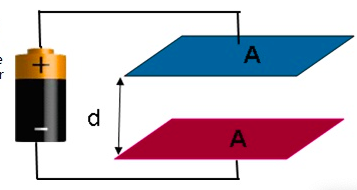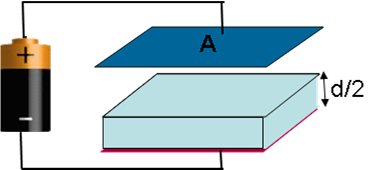# Problem: Two parallel plates, each having area A = 2312 cm2 are connected to the terminals of a battery voltage Vb = 6V as shown. The plates are separated by a distance d = 0.46 cm. You may assume (contrary to the drawing) that the separation between the plates is small compared to a linear dimension of the plate.1. What is C, the capacitance of this parallel plate capacitor?2) What is Q, the charge stored on the top plate of this capacitor?.3) A dielectric having a dielectric constant? = 4.4 is now inserted in between the plates of the capacitor as shown. The dielectric has area A = 2312 cm2 and thickness equal to half of the separation (= 0.23 cm). What is the charge on the top plate of this capacitor?4) What is U, the energy stored in this capacitor?5) The battery is now disconnected from the capacitor and then the dielectric is withdrawn. What is V, the voltage across the capacitor?

###### FREE Expert Solution

This problem involves capacitance, potential difference, and stored charge.

We'll need the following equations.

Capacitance:

87% (430 ratings)###### Problem Details

Two parallel plates, each having area A = 2312 cm2 are connected to the terminals of a battery voltage Vb = 6V as shown. The plates are separated by a distance d = 0.46 cm. You may assume (contrary to the drawing) that the separation between the plates is small compared to a linear dimension of the plate.1. What is C, the capacitance of this parallel plate capacitor?

2) What is Q, the charge stored on the top plate of this capacitor?.

3) A dielectric having a dielectric constant? = 4.4 is now inserted in between the plates of the capacitor as shown. The dielectric has area A = 2312 cm2 and thickness equal to half of the separation (= 0.23 cm). What is the charge on the top plate of this capacitor?4) What is U, the energy stored in this capacitor?

5) The battery is now disconnected from the capacitor and then the dielectric is withdrawn. What is V, the voltage across the capacitor?